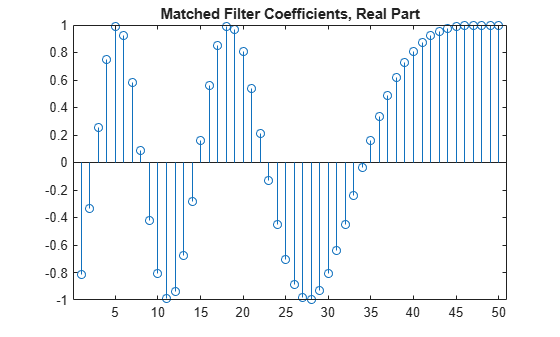# getMatchedFilter

Matched filter coefficients derived from waveform

Since R2023a

## Syntax

``coeff = getMatchedFilter(waveform)``
``coeff = getMatchedFilter(waveform,FrequencyOffset = foffset)``

## Description

example

````coeff = getMatchedFilter(waveform)` returns the matched filter coefficients `coeff` for the pulse FM `waveform` object.```
````coeff = getMatchedFilter(waveform,FrequencyOffset = foffset)` also specifies a frequency offset `foffset` when matched filter coefficients are generated. To enable this syntax, set the `FrequencyOffsetSource` property of the `waveform` to `'Input port'`.```

## Examples

collapse all

Get the matched filter coefficients for a linear FM pulse.

```waveform = phased.LinearFMWaveform('PulseWidth',5e-05,... 'SweepBandwidth',1e5,'OutputFormat','Pulses'); coeff = getMatchedFilter(waveform); stem(real(coeff)) title('Matched Filter Coefficients, Real Part')```## Input Arguments

collapse all

Pulse waveform, specified as a waveform System object.

Example: `phased.RectangularWaveform`

Frequency offset, specified as a scalar. Units are in Hz.

Example: `12000.0`

Data Types: `double`

## Output Arguments

collapse all

Matched filter coefficients, returned as a complex-valued column vector. Units are dimensionless.

## Version History

Introduced in R2023a